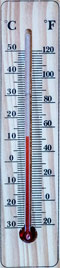# Celsius to Fahrenheit

 From Celsius (°C) Fahrenheit (°F) Kelvin (K) To Celsius (°C) Fahrenheit (°F) Kelvin (K) °C = ? °FThis converter makes instant conversions from Celsius (°C) to Fahrenheit (°F). It also provides the conversion steps. To use the converter, please provide a value in the input field. The converter will begin making conversions as the values are being typed in.

A Celsius is a unit of temperature on the Celsius scale. Along with the degree Kelvin, it is one of the two units of temperature used within the International System of Units (SI). The Fahrenheit is the unit of temperature in the Fahrenheit scale. Although it is not used within SI, it is still widely used within the United States and some other countries. In most other countries around the world, the Celsius is the officially adopted measurement of temperature.

### How to convert Celsius to Fahrenheit

Historically, the definitions of both the Celsius and the Fahrenheit scales were based on the freezing and boiling points of water. In the Celsius scale, the freezing point was 0°C and the boiling point was 100°C at 1 atm pressure. In the Fahrenheit scale, these two points were defined to be 32°F and 212°F. Based on these two definitions, the Celsius to Fahrenheit conversion formula is:

°F =
 9 5
× °C + 32

For example, a body temperature of 37.5°C can be converted from Celsius to Fahrenheit as:

37.5°C = (
 9 5
× 37.5 + 32)°F = 99.5°F

Even though the definitions of both Celsius and Fahrenheit have changed as scientific understandings evolve, the relationship between the Celsius and Fahrenheit scales remains the same, so the conversion formula above still holds.

### Celsius to Fahrenheit chart

The following chart provides a quick reference of various Celsius to Fahrenheit conversions:

CelsiusFahrenheitCelsiusFahrenheit
-50°C-58°F39°C102.2°F
-20°C-4°F40°C104°F
-10°C14°F41°C105.8°F
0°C32°F50°C122°F
10°C50°F100°C212°F
20°C68°F175°C347°F
25°C77°F200°C392°F
30°C86°F220°C428°F
37°C98.6°F500°C932°F
38°C100.4°F1000°C1832°F